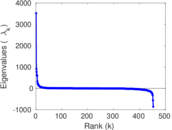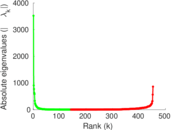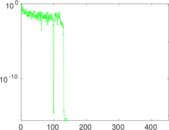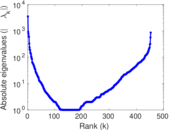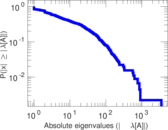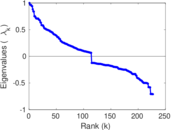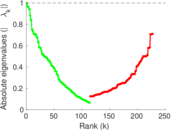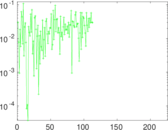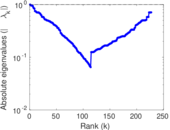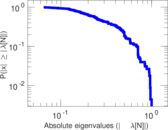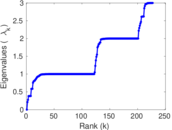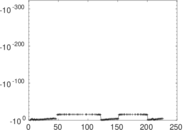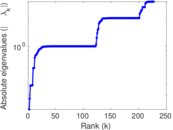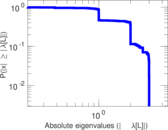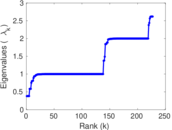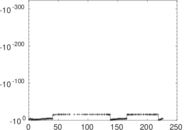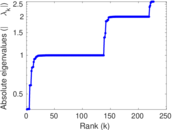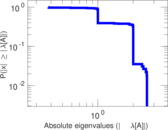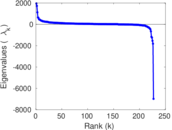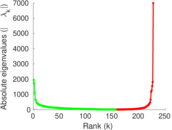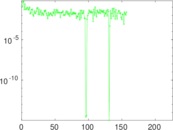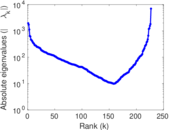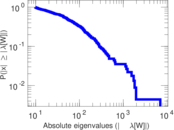# DNC co-recipients

This is the undirected network of people having received the same email in the 2016 Democratic National Committee email leak. The Democratic National Committee (DNC) is the formal governing body for the United States Democratic Party. A dump of emails of the DNC was leaked in 2016, and this dataset contains persons from that dump as nodes, and an edge when two persons received the same email, i.e., when two persons were on the recipient list of the same email. Multiple edges indicate multiple emails.

 Code `DN` Internal name `dnc-corecipient` Name DNC co-recipients Data source http://www.rene-pickhardt.de/extracting-2-social-network-graphs-from-the-democratic-national-committee-email-corpus-on-wikileaks/ AvailabilityDataset is available for download Consistency checkDataset passed all tests Category Online contact network Dataset timestamp 2016 Node meaning Person Edge meaning Co-recipientship Network format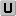Unipartite, undirected Edge typeUnweighted, multiple edges LoopsDoes not contain loops Snapshot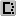Is a snapshot and likely to not contain all data Join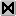Is the join of an underlying network

## Statistics

 Size n = 2,029 Volume m = 136,602 Unique edge count m̿ = 12,085 Loop count l = 0 Wedge count s = 955,910 Claw count z = 86,121,142 Cross count x = 5,511,669,921 Triangle count t = 174,677 Square count q = 10,408,369 4-Tour count T4 = 87,111,450 Maximum degree dmax = 462 Average degree d = 134.650 Fill p = 0.029 478 1 Average edge multiplicity m̃ = 11.303 4 Size of LCC N = 849 Diameter δ = 8 50-Percentile effective diameter δ0.5 = 2.204 17 90-Percentile effective diameter δ0.9 = 3.306 08 Median distance δM = 3 Mean distance δm = 2.713 72 Gini coefficient G = 0.889 309 Balanced inequality ratio P = 0.109 475 Relative edge distribution entropy Her = 0.842 080 Power law exponent γ = 1.583 17 Tail power law exponent γt = 1.451 00 Tail power law exponent with p γ3 = 1.451 00 p-value p = 0.000 00 Degree assortativity ρ = −0.124 892 Degree assortativity p-value pρ = 2.796 50 × 10−73 Clustering coefficient c = 0.548 201 Spectral norm α = 3,522.12 Algebraic connectivity a = 0.211 770 Spectral separation |λ1[A] / λ2[A]| = 3.867 82 Non-bipartivity bA = 0.755 203 Normalized non-bipartivity bN = 0.274 849 Algebraic non-bipartivity χ = 0.368 608 Spectral bipartite frustration bK = 0.003 767 20 Controllability C = 283 Relative controllability Cr = 0.312 362

## Plots

### Fruchterman–Reingold graph drawing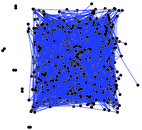### Degree distribution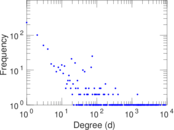### Cumulative degree distribution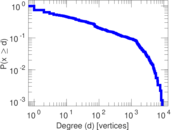### Lorenz curve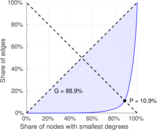### Spectral distribution of the adjacency matrix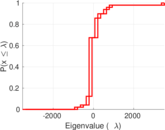### Spectral distribution of the normalized adjacency matrix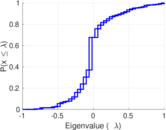### Spectral distribution of the Laplacian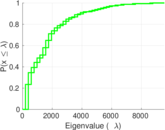### Spectral graph drawing based on the adjacency matrix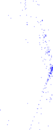### Spectral graph drawing based on the Laplacian### Spectral graph drawing based on the normalized adjacency matrix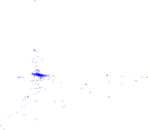### Degree assortativity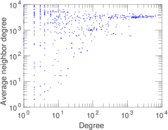### Zipf plot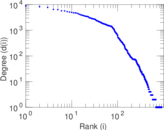### Hop distribution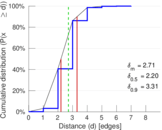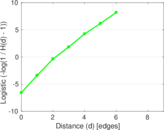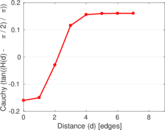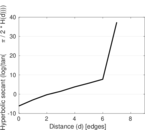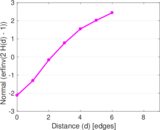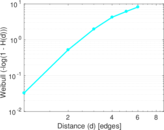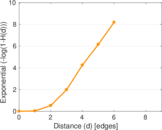### Double Laplacian graph drawing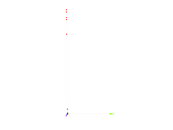### Delaunay graph drawing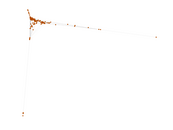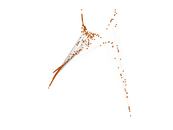### Edge weight/multiplicity distribution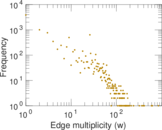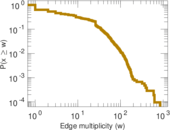### Clustering coefficient distribution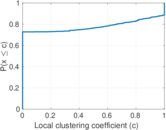### Average neighbor degree distribution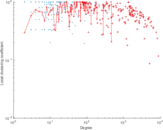### SynGraphy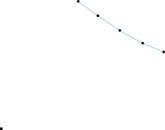### Matrix decompositions plots